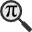## General

Display information for equation id:math.286743.22 on revision:286743

* Page found: Talk:Covariance matrix (eq math.286743.22)

(force rerendering)

Cannot find the equation data in the database. Fetching from revision text.

Occurrences on the following pages:

Hash: c3198a6dbef629ca31403b4ccdff3fc7

TeX (original user input):

2\pi


TeX (checked):

2\pi


### LaTeXML (experimental; uses MathML) rendering

MathML (875 B / 340 B) :$2\pi$
<math xmlns="http://www.w3.org/1998/Math/MathML" id="p1.1.m1.1" class="ltx_Math" alttext="{\displaystyle 2\pi}" display="inline">
<semantics id="p1.1.m1.1a">
<mrow id="p1.1.m1.1.3" xref="p1.1.m1.1.3.cmml">
<mn id="p1.1.m1.1.1" xref="p1.1.m1.1.1.cmml">2</mn>
<mo id="p1.1.m1.1.3.1" xref="p1.1.m1.1.3.1.cmml">⁢</mo>
<mi id="p1.1.m1.1.2" xref="p1.1.m1.1.2.cmml">π</mi>
</mrow>
<annotation-xml encoding="MathML-Content" id="p1.1.m1.1b">
<apply id="p1.1.m1.1.3.cmml" xref="p1.1.m1.1.3">
<times id="p1.1.m1.1.3.1.cmml" xref="p1.1.m1.1.3.1"/>
<cn type="integer" id="p1.1.m1.1.1.cmml" xref="p1.1.m1.1.1">2</cn>
<ci id="p1.1.m1.1.2.cmml" xref="p1.1.m1.1.2">π</ci>
</apply>
</annotation-xml>
<annotation encoding="application/x-tex" id="p1.1.m1.1c">{\displaystyle 2\pi}</annotation>
</semantics>
[/itex]


SVG image empty. Force Re-Rendering

SVG (1.795 KB / 947 B) :

### MathML with SVG or PNG fallback (recommended for modern browsers and accessibility tools) rendering

SVG image empty. Force Re-Rendering

SVG (0 B / 8 B) :

PNG (0 B / 8 B) :

$2\pi$## Translations to Computer Algebra Systems

### Translation to Maple

In Maple: 2*pi

\pi: Could be the ratio of a circle's circumference to its diameter == Archimedes' constant.

But it is also a Greek letter. Be aware, that this program translated the letter as a normal Greek letter and not as a constant!

Use the DLMF-Macro \cpi to translate \pi as a constant.

### Translation to Mathematica

In Mathematica: 2*\[Pi]

\pi: Could be the ratio of a circle's circumference to its diameter == Archimedes' constant.

But it is also a Greek letter. Be aware, that this program translated the letter as a normal Greek letter and not as a constant!

Use the DLMF-Macro \cpi to translate \pi as a constant.

## Similar pages

Calculated based on the variables occurring on the entire Talk:Covariance matrix page

## Identifiers

• $\pi$### MathML observations

0results

0results

no statistics present please run the maintenance script ExtractFeatures.php

0 results

0 results